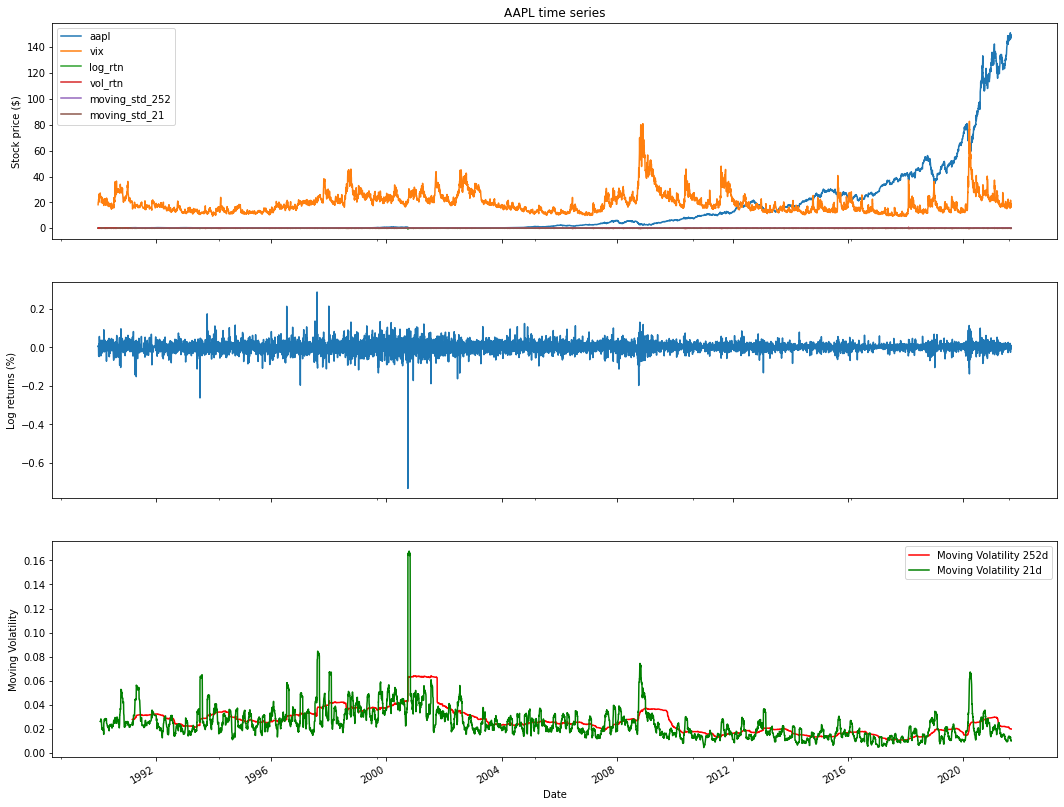# Stock price APPLE with python

## Apple is an American company of electronics and software. It is one of the largest electronic companies.

Analyzing Apple Inc.'s stock price with Python involves collecting historical price data, performing data analysis, and creating visualizations to gain insights into the company's stock performance. Here's a step-by-step guide on how to conduct Apple stock price analysis in Python:

Import Libraries:
Start by importing the necessary Python libraries for data manipulation, analysis, and visualization. Commonly used libraries include pandas, numpy, matplotlib, and yfinance to fetch historical data:

Python
Copy code
import pandas as pd
import numpy as np
import matplotlib.pyplot as plt
import yfinance as yf
Data Retrieval:
Use the yfinance library or other financial data sources to fetch historical data for Apple's stock. Specify the start and end dates for the data you want to analyze:

Python
Copy code
Data Exploration:
Explore the fetched data to understand its structure and contents. Use functions like head(), tail(), describe(), and info() to inspect the dataset:

Python
Copy code
Data Visualization:
Create visualizations to analyze the historical performance of Apple's stock. Common visualizations include line charts to visualize price movements:

Python
Copy code
plt.figure(figsize=(12, 6))
plt.title('Apple Stock Price')
plt.xlabel('Date')
plt.ylabel('Price')
plt.legend()
plt.show()
Technical Analysis (Optional):
Perform technical analysis by calculating and visualizing technical indicators like moving averages, relative strength index (RSI), and MACD. Libraries like ta-lib can be used for these calculations.

Statistical Analysis (Optional):
Conduct statistical analysis to calculate summary statistics, volatility measures, and correlations with other assets. numpy and pandas are useful for these calculations.

Sentiment Analysis (Optional):
Consider incorporating sentiment analysis of news articles or social media data related to Apple to understand market sentiment's impact on the stock price.

Fundamental Analysis (Optional):
Analyze fundamental factors affecting Apple, such as earnings reports, revenue growth, product launches, and market share, which can influence the stock's performance.

Prediction and Forecasting (Optional):
You can use time series forecasting techniques like ARIMA or machine learning models to make predictions about future Apple stock price movements.

Risk Management and Decision Making:
Based on your analysis, formulate investment strategies, set risk management parameters, and make informed investment decisions regarding Apple's stock.

Keep your analysis up to date with the latest data to adapt to changing market conditions and make timely decisions.

Remember that investing in stocks carries risks, and it's crucial to do thorough research, consider factors like company news and market trends, and potentially consult with financial experts before making investment decisions based on your analysis of Apple's stock price or any other stock.
How to get Apple stock price data in Python?
!pip install yfinance

### How to get financial data from Yahoo Finance in Python?

import pandas as pd
import numpy as np
import yfinance as yf
start='2020-01-01',
end='2021-08-28',
progress=False)
df.tail(9)
Date
2021-08-17150.229996151.679993149.089996150.190002150.19000292229700
2021-08-18149.800003150.720001146.149994146.360001146.36000186326000
2021-08-19145.029999148.000000144.500000146.699997146.69999786960300
2021-08-20147.440002148.500000146.779999148.190002148.19000259947400
2021-08-23148.309998150.190002147.889999149.710007149.71000760131800
2021-08-24149.449997150.860001149.149994149.619995149.61999548606400
2021-08-25149.809998150.320007147.800003148.360001148.36000158991300
2021-08-26148.350006149.119995147.509995147.539993147.53999348597200
2021-08-27147.479996148.750000146.830002148.600006148.60000655721500

### There is a number of alternative sources like Quandal,intrinio,google others.

import matplotlib.pyplot as plt

### How to plot Apple close price?

df['Close'].plot(figsize=(12,8))
<matplotlib.axes._subplots.AxesSubplot at 0x7f6c0d5e2510>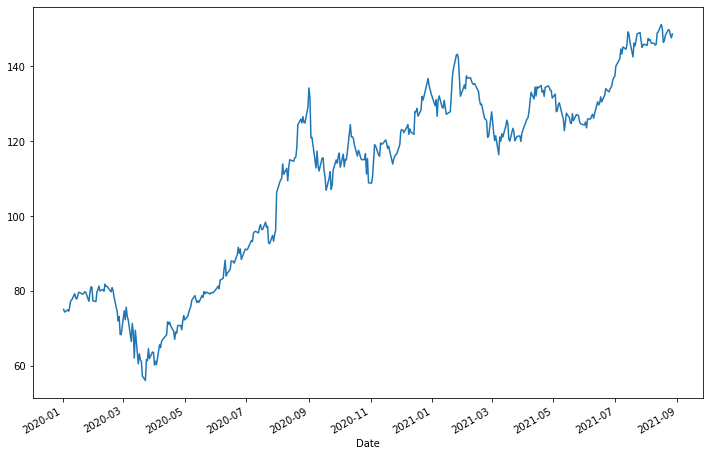### How to plot Apple close price and volume?

df[['Close''Volume']].plot(subplots=True, style='b',
figsize=(128))
array([<matplotlib.axes._subplots.AxesSubplot object at 0x7f6c0d4c9f50>,
<matplotlib.axes._subplots.AxesSubplot object at 0x7f6c0d049690>],
dtype=object)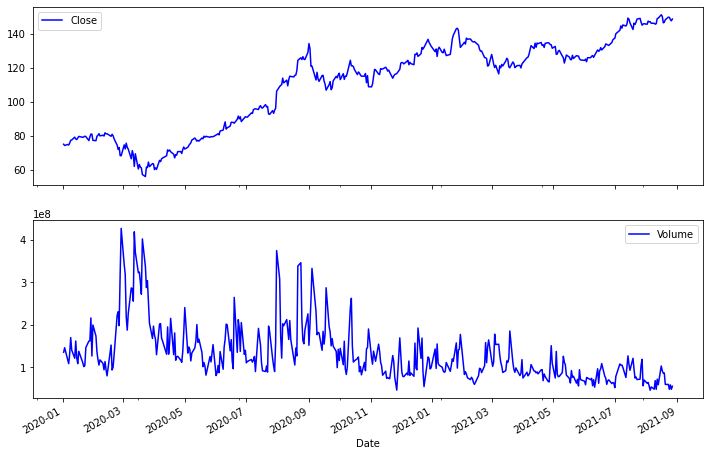df.describe()

### How to convert Apple Stock prices into logs and simple returns in Python?

df['simple_rtn'] = df.Close.pct_change()
df['log_rtn'] = np.log(df.Close/df.Close.shift(1))
df['log_rtn'].plot(subplots=True, style='b',
figsize=(128))
array([<matplotlib.axes._subplots.AxesSubplot object at 0x7f6c0cf3bf50>],
dtype=object)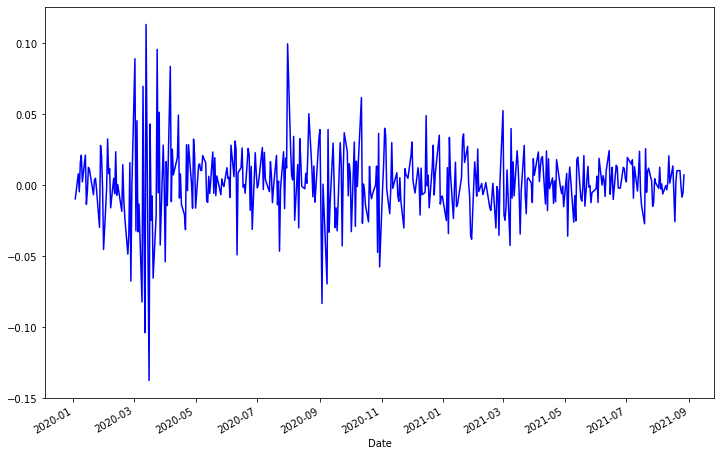df['log_rtn'].tail(12)
Date 2021-08-12 0.020561 2021-08-13 0.001409 2021-08-16 0.013457 2021-08-17 -0.006173 2021-08-18 -0.025832 2021-08-19 0.002320 2021-08-20 0.010106 2021-08-23 0.010205 2021-08-24 -0.000601 2021-08-25 -0.008457 2021-08-26 -0.005542 2021-08-27 0.007159 Name: log_rtn, dtype: float64
from plotly.offline import iplot, init_notebook_mode
init_notebook_mode()
df_rolling = df[['simple_rtn']].rolling(window=21) \
.agg(['mean''std'])
df_rolling.columns = df_rolling.columns.droplevel()
df_outliers = df.join(df_rolling)
import pandas as pd
import numpy as np
import yfinance as yf
import seaborn as sns
import scipy.stats as scs
import statsmodels.api as sm
import statsmodels.tsa.api as smt

### How to compare Apple stock price and Vix?

start='1985-01-01',
end='2021-08-28',
progress=False)
df.columns = df.columns.droplevel(0)
df = df.rename(columns={'AAPL''aapl''^VIX''vix'})
df.tail()
df['log_rtn'] = np.log(df.aapl / df.aapl.shift(1))
df['vol_rtn'] = np.log(df.vix / df.vix.shift(1))
df.dropna(how='any', axis=0, inplace=True)
corr_coeff = df.log_rtn.corr(df.vol_rtn)
corr_coeff = df.log_rtn.corr(df.vol_rtn)
ax = sns.regplot(x='log_rtn', y='vol_rtn', data=df,
line_kws={'color''red'})
ax.set(title=f'AAPL vs. VIX ($\\rho$ = {corr_coeff:.2f})',
ylabel='VIX log returns',
xlabel='AAPL log returns')
[Text(0, 0.5, 'VIX log returns'),
Text(0.5, 0, 'AAPL log returns'),
Text(0.5, 1.0, 'AAPL vs. VIX ($\\rho$ = -0.34)')]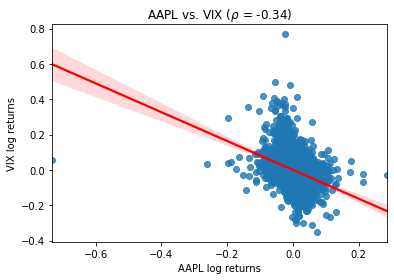r_range = np.linspace(min(df.log_rtn), max(df.log_rtn), num=1000)
mu = df.log_rtn.mean()
sigma = df.log_rtn.std()
norm_pdf = scs.norm.pdf(r_range, loc=mu, scale=sigma)
fig, ax = plt.subplots(12, figsize=(168))
# histogram
sns.distplot(df.log_rtn, kde=False, norm_hist=True, ax=ax)
ax.set_title('Distribution of AAPL returns', fontsize=16)
ax.plot(r_range, norm_pdf, 'g', lw=2,
label=f'N({mu:.2f}{sigma**2:.4f})')
ax.legend(loc='upper left');

### How to plot apple distribution of stock price and Q-Q plot?

# Q-Q plot
qq = sm.qqplot(df.log_rtn.values, line='s', ax=ax)
ax.set_title('Q-Q plot', fontsize = 16)
/usr/local/lib/python3.7/dist-packages/seaborn/distributions.py:2557: FutureWarning:

distplot is a deprecated function and will be removed in a future version. Please adapt your code to use either displot (a figure-level function with similar flexibility) or histplot (an axes-level function for histograms).


Text(0.5, 1.0, 'Q-Q plot')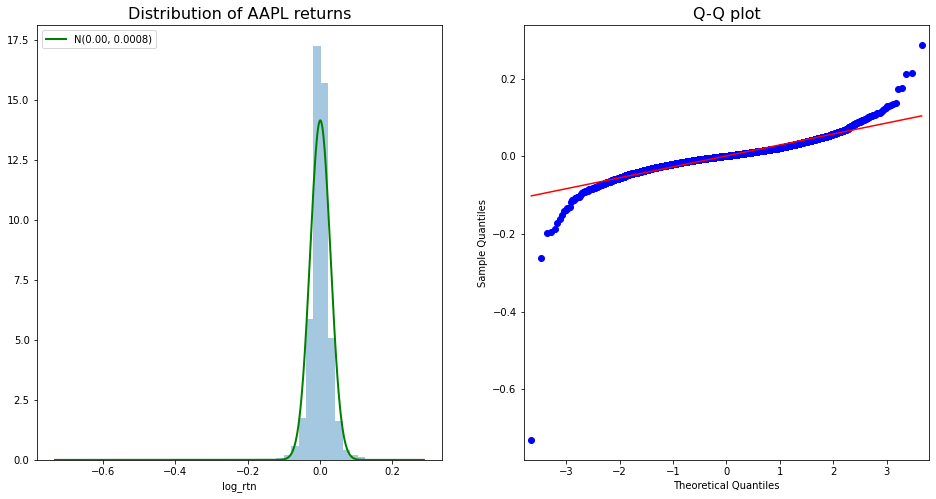### How to plot Apple daily stock return?

df.log_rtn.plot(title='Daily AAPL returns')
<matplotlib.axes._subplots.AxesSubplot at 0x7f6be20b5fd0>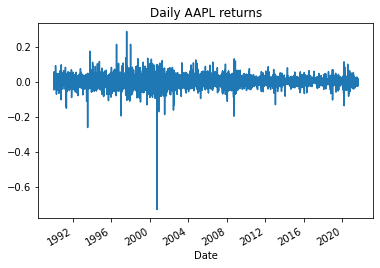N_LAGS = 50
SIGNIFICANCE_LEVEL = 0.05
acf = smt.graphics.plot_acf(df.log_rtn,
lags=N_LAGS,
alpha=SIGNIFICANCE_LEVEL
)

### How to plot apple absolute return and autocorrelation?

fig, ax = plt.subplots(21, figsize=(1210))
smt.graphics.plot_acf(df.log_rtn ** 2, lags=N_LAGS,
alpha=SIGNIFICANCE_LEVEL, ax = ax)
ax.set(title='Autocorrelation Plots',
ylabel='Squared Returns')
smt.graphics.plot_acf(np.abs(df.log_rtn), lags=N_LAGS,
alpha=SIGNIFICANCE_LEVEL, ax = ax)
ax.set(ylabel='Absolute Returns',
xlabel='Lag')
[Text(0, 0.5, 'Absolute Returns'), Text(0.5, 0, 'Lag')]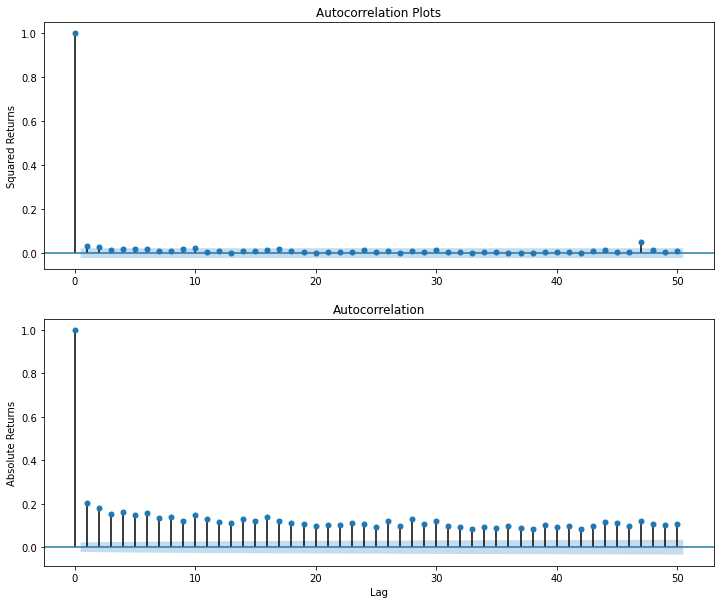### How to plot Apple's 252 days and 21 days moving volatility?

df['moving_std_252'] = df[['log_rtn']].rolling(window=252).std()
df['moving_std_21'] = df[['log_rtn']].rolling(window=21).std()
fig, ax = plt.subplots(31, figsize=(1815),
sharex=True)
df.plot(ax=ax)
ax.set(title='AAPL time series',
ylabel='Stock price (\$)')
df.log_rtn.plot(ax=ax)
ax.set(ylabel='Log returns (%)')
df.moving_std_252.plot(ax=ax, color='r',
label='Moving Volatility 252d')
df.moving_std_21.plot(ax=ax, color='g',
label='Moving Volatility 21d')
ax.set(ylabel='Moving Volatility',
xlabel='Date')
ax.legend()
<matplotlib.legend.Legend at 0x7f6be1b47fd0>